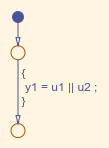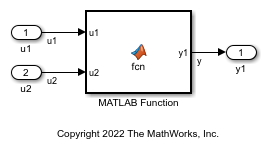Relational and Logical Operators

This example shows how to implement relational and logical operators by using Simulink blocks, Stateflow Charts, and MATLAB Function blocks.

Modeling Pattern for Relational or Logical Operators — Simulink Blocks

To include a logical operation in your model, use the Logical Operator block from the Logic and Bit Operations library.

1. Open example model ex_data_type_SL.The Logical Operator block performs an OR operation in the model. To change the operation, double-click the block and set the Operator field to any of the operations in the menu.

You can implement relational operators by replacing the Logical Operator block with a Relational Operator block.

2. To build the model and generate code, press Ctrl+B.

The code implementing the logical operator OR is in the ex_logical_SL_step function in ex_logical_SL.c.

/* Exported block signals */
boolean_T u1;                          /* '<Root>/u1' */
boolean_T u2;                          /* '<Root>/u2' */
boolean_T y1;                          /* '<Root>/y1' */

/* Model step function */
void ex_logical_SL_step(void)
{
/* Outport: '<Root>/y1' incorporates:
*  Inport: '<Root>/u1'
*  Inport: '<Root>/u2'
*  Logic: '<Root>/Logical Operator'
*/
y1 = (u1 || u2);
}

Modeling Pattern for Relational and Logical Operators — Stateflow Chart

1. Open example model ex_data_type_SF.In the Stateflow chart, the relational or logical operation actions are on the transition from one junction to another. Relational statements specify conditions to conditionally allow a transition. In that case, the statements are within square brackets.

2. To build the model and generate code, press Ctrl+B.

The code implementing the logical operator OR is in the ex_logical_SF_step function in ex_logical_SF.c.

/* Exported block signals */
boolean_T u1;                          /* '<Root>/u1' */
boolean_T u2;                          /* '<Root>/u2' */
boolean_T y1;                          /* '<Root>/Logical Operator' */

/* Model step function */
void ex_logical_SF_step(void)
{
/* Chart: '<Root>/Logical Operator' incorporates:
*  Inport: '<Root>/u1'
*  Inport: '<Root>/u2'
*/
y1 = (u1 || u2);
}

Modeling Pattern for Relational and Logical Operators — MATLAB Function Block

This example shows the MATLAB Function block method for incorporating operators into the generated code by using a relational operator.

1. Open example model ex_logical_ML.2. The MATLAB Function Block contains this function:

function y1 = fcn(u1, u2)
y1 = u1 > u2;
end

3. To build the model and generate code, press Ctrl+B.

The generated code appears in ex_data_type_ML.c:

/* Exported block signals */
real_T u1;                             /* '<Root>/u1' */
real_T u2;                             /* '<Root>/u2' */
boolean_T y;                           /* '<Root>/MATLAB Function' */

/* Model step function */
void ex_logical_ML_step(void)
{
/* MATLAB Function: '<Root>/MATLAB Function' incorporates:
*  Inport: '<Root>/u1'
*  Inport: '<Root>/u2'
*/
y = (u1 > u2);
}